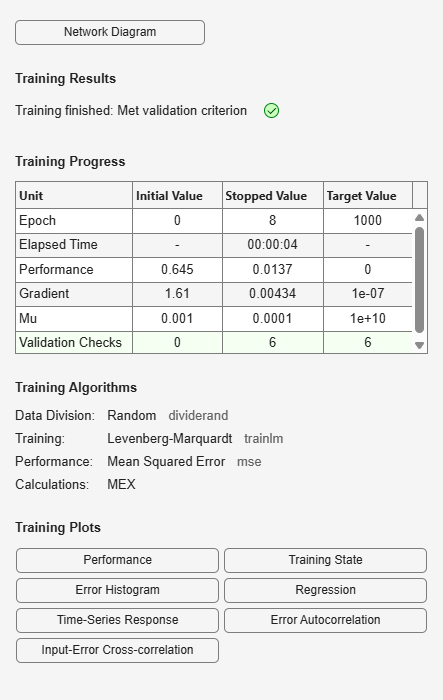Documentation

distdelaynet

Distributed delay network

Syntax

distdelaynet(delays,hiddenSizes,trainFcn)

Description

Distributed delay networks are similar to feedforward networks, except that each input and layer weights has a tap delay line associated with it. This allows the network to have a finite dynamic response to time series input data. This network is also similar to the time delay neural network (timedelaynet), which only has delays on the input weight.

distdelaynet(delays,hiddenSizes,trainFcn) takes these arguments,

 delays Row vector of increasing 0 or positive delays (default = 1:2) hiddenSizes Row vector of one or more hidden layer sizes (default = 10) trainFcn Training function (default = 'trainlm')

and returns a distributed delay neural network.

Examples

Distributed Delay Network

Here a distributed delay neural network is used to solve a simple time series problem.

[X,T] = simpleseries_dataset;
net = distdelaynet({1:2,1:2},10);
[Xs,Xi,Ai,Ts] = preparets(net,X,T);
net = train(net,Xs,Ts,Xi,Ai);
view(net)
Y = net(Xs,Xi,Ai);
perf = perform(net,Y,Ts)
perf =

0.0323